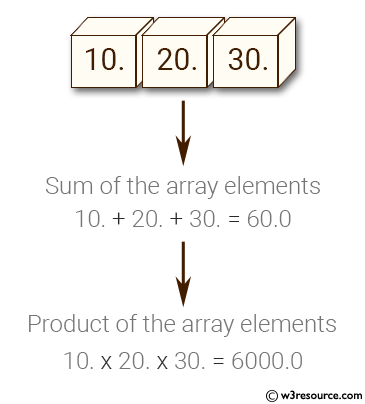﻿ NumPy: Sum and compute the product of a NumPy array elements - w3resource# NumPy: Sum and compute the product of a NumPy array elements

## NumPy: Array Object Exercise-99 with Solution

Write a NumPy program to sum and compute the product of a NumPy array elements.

Pictorial Presentation:Sample Solution:-

Python Code:

``````import numpy as np
x = np.array([10, 20, 30], float)
print("Original array:")
print(x)
print("Sum of the array elements:")
print(x.sum())
print("Product of the array elements:")
print(x.prod())
```
```

Sample Output:

```Original array:
[ 10.  20.  30.]
Sum of the array elements:
60.0
Product of the array elements:
6000.0
```

Python Code Editor:

Have another way to solve this solution? Contribute your code (and comments) through Disqus.

What is the difficulty level of this exercise?

Test your Python skills with w3resource's quiz

﻿

## Python: Tips of the Day

List comprehension:

```>>> m = [x ** 2 for x in range(5)]
>>> m
[0, 1, 4, 9, 16]
```